# What is the smallest number of disjoint spanning trees made a graph Hamiltonian

 Importance: Medium ✭✭
 Author(s): Goldengorin
 Subject: Graph Theory » Extremal Graph Theory
 Keywords: 1-trees cycle Hamitonian path spanning trees
 Posted by: boris on: September 10th, 2007

We are given a complete simple undirected weighted graph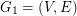and its first arbitrary shortest spanning tree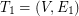. We define the next graph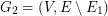and find onthe second arbitrary shortest spanning tree. We continue similarly by findingon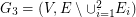, etc. Let k be the smallest number of disjoint shortest spanning trees as defined above and letbe the graph obtained as union of alldisjoint trees.

Question 1. What is the smallest number of disjoint spanning trees creates a graphcontaining a Hamiltonian path.

Question 2. What is the smallest number of disjoint spanning trees creates a graphcontaining a shortest Hamiltonian path?

Questions 3 and 4. Replace in questions 1 and 2 a shortest spanning tree by a 1-tree. What is the smallest number of disjoint 1-trees creates a Hamiltonian graph? What is the smallest number of disjoint 1-trees creates a graph containing a shortest Hamiltonian cycle?

These questions are induced by the following paper Chrobak and Poljak. On common edges in optimal solutions to travelling salesman and other optimization problems, Discrete Applied Mathematics 20 (1988) 101-111.

## Bibliography

M. Chrobak and S. Poljak. On common edges in optimal solutions to travelling salesman and other optimization problems, Discrete Applied Mathematics 20 (1988) 101-111.

* indicates original appearance(s) of problem.

### Is this statement correct?

Unless I am mistaken, it appears that there does not exist anyfor which any of the above problems has a positive solution. For instance, letbe the complete graph with vertex set, and define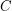to be the edge-cut ofconsisting of all edges between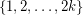and. Now define a weighting ofby assigning each edge inweight 1 and every other edge weight 2. So, the subgraph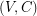(consisting of all edges of weight 1) is isomorphic to- and sincehasedge-disjoint spanning trees (by the Nash-Williams theorem, say), our procedure may well choose treesso that all of the edges in all of these graphs are in. But thenwill not have a Hamiltonian path since it is a subgraph of.

Of course, we may modify the edge weights here so that the procedure is forced to choose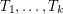so that all of these trees have their edges in.